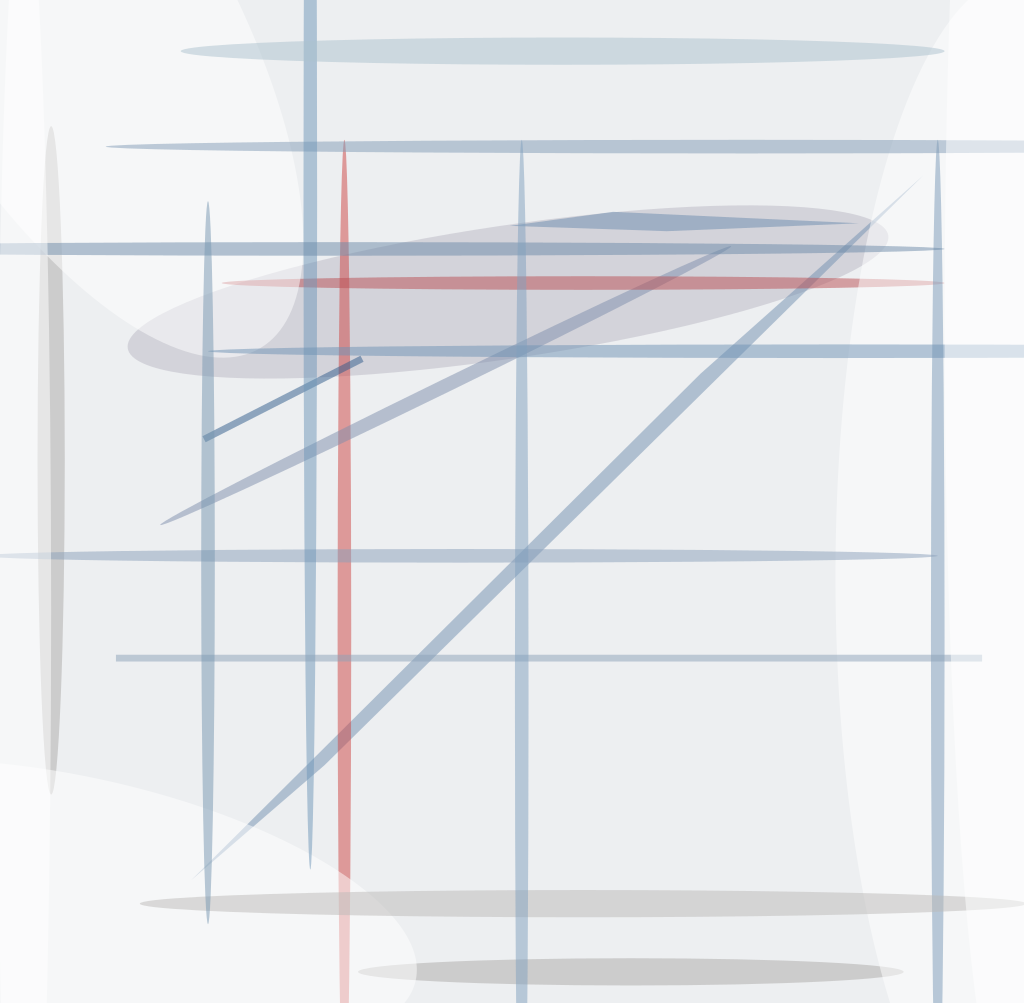In discussing fatigue life, it is important to distinguish between disc springs and conical spring washers, as they differ by design and intended usage. Conical spring washers are designed to provide a static thrust load in bolted joints and should not be used in fatigue applications; they are specified by DIN 6796.

Disc springs may be used to apply either static or dynamic loads and are specified by DIN EN 16983 (formerly DIN 2093). Typically, disc springs have a thinner cross-section than conical spring washers. Some variation in size is permissible, but calculations only apply to spring steels and when the ratio of the outside diameter (OD) to thickness is between 16 and 40, and the ratio of OD to inside diameter (ID) is between 1.8 and 2.5. Deflection of a disc spring at a given load is predictable, making it possible to calculate force and stress levels in the disc. As the disc spring flexes, stress levels in the disc change; the greater the change, the faster the disc spring fatigues.

Tensile stress at points II and III in Figure 1 are critical in determining fatigue life. These locations are where fatigue cracks originate. Estimation of fatigue life requires evaluation of the maximum stress difference between preload and final load at locations II and III. The location with the highest stress differential is used to estimate fatigue life. Once it is determined which stress values will be used (from location II or III), the fatigue life charts in DIN EN 16983 can be used to estimate the fatigue life of the disc spring. Stress values may be found in the disc spring product catalog, DIN EN 16983, or calculated using formulas in DIN EN 16984. Fatigue life charts are provided in three thickness ranges: <1.25 mm, between 1.25 and 6 mm, and between 6 and 14 mm. The following examples explain how to interpret the fatigue life charts.

The first example estimates the fatigue life of a DIN EN 16983 Series B Group 2, DSC 50 × 25.4 × 2 disc spring with a preload of 15% of its initial height with a final position at 75% of its initial height. Using the specification chart in Figure 2, Stress II (σII) at 15% is 128 N/mm2 and Stress III (σIII) is 264 N/mm2. Stress II (σII) at 75% is 923 N/mm2 and Stress III (σIII) is 1,140 N/mm2. Now the differences between the stress at each location can be calculated.

923 N/mm2 – 128 N/mm2 = 795 N/mm2 Stress II at 75% Stress II at 15%

1,140 N/mm2 – 264 N/mm2 = 876 N/mm2 Stress III at 75% Stress III at 15%

As seen in the calculation above, the maximum differential in stress occurs at location III; therefore, stress values from location III and the fatigue life charts can be used to estimate the fatigue life of the disc spring.Figure 3. Estimated fatigue life for DSC 50 x 25.4 x 2 disc spring in Example 1.

The intersection of a vertical line drawn on the X-axis representing the minimum stress at location III and a horizontal line drawn on the Y-axis representing the maximum stress at location III is the estimated fatigue life. In this example and using Figure 3, the line on the X-axis is drawn at 264 N/mm2 and the line drawn on the Y-axis is drawn at 1,140 N/mm2. The intersection is slightly above the “100,000 cycle” line as represented by N = 105. This represents an estimated fatigue life of slightly less than 100,000 cycles.